Arduino Alarm Clock Project | vilros.com – Vilros.com

# Arduino Alarm Clock Project

### Alarm Clock Project

In this project, we will use the Arduino Uno to control an LCD screen to show the current time and time that an alarm is set for. We will use buttons to set each time.

### Hookup Instructions

1. #### Connect a jumper wire from the 5V pin on the Arduino to one of the + rails on the breadboard.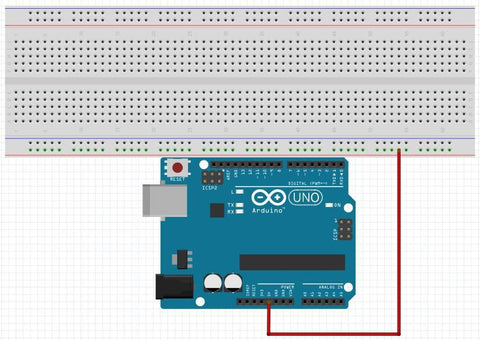1. #### Connect a jumper wire from the GND pin on the Arduino to the - rail next to the + rail you chose on the breadboard.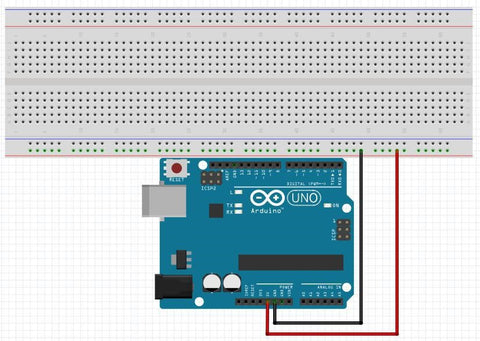1. #### Connect the LCD screen to power, ground, and the TX pin (pin 1).1. #### Place 7 buttons on the breadboard with the legs across the gap in the breadboard.1. #### Place 10 kohm resistors from the - rail with the GND pin connected to it to the bottom left pins of the buttons.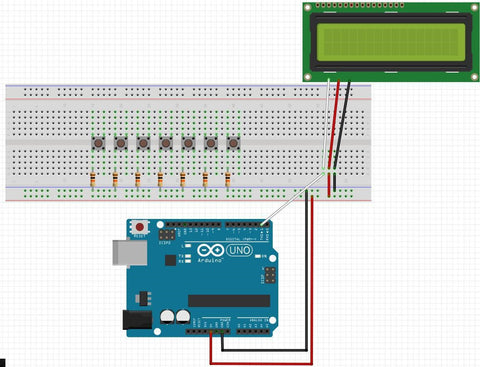1. #### Place jumper wires between the bottom right pin of the buttons and the 5V rail on your breadboard.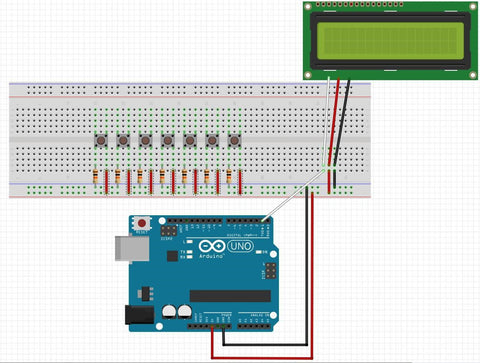1. #### Place jumper wires between pins 6, then 8-13, and the pin on the button that the resistor is connected to.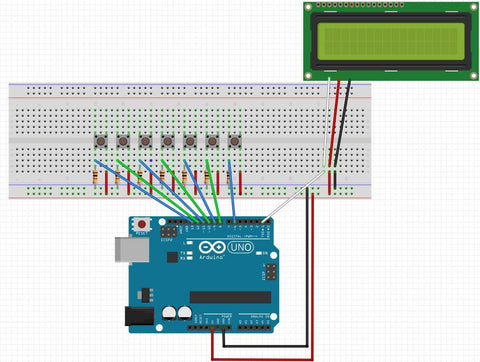1. #### Next, place your Piezo speaker on the breadboard and connect pin 7 to the power pin, then a 100 ohm resistor to ground.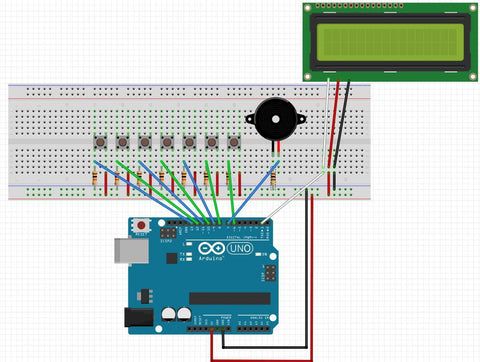### Programming Instructions

1. Overview: This project will ask the user to set the current time on initial power on the display the current time and the time the alarm is set for. The buttons connected above will be used to set each time. From left to right, they are set current hour, set current minute, set current AM or PM, set alarm hour, set alarm minute, set alarm AM or PM. The last button is used to silence the alarm when it’s sounding.
2. The first thing we need to do is initialize our variable we’re going to use.

// Initialize variables to be used

int hour = 0; // Hour for current time int minute = 0; //

Minute for current time int second = 0; // Second for current time

int hour_a = 0; int              // Hour for alarm time

minute_a = 0;                   // Minute for alarm time

bool am_pm = false;   // AM/PM toggle flag.   False is AM, True is PM

bool am_pm_a = false; // AM/PM toggle flag for alarm. False is AM, True is PM

int set_hr = 13;                    // Use pin 13 to set hour

int set_min = 12; // Use pin 12 to set minute int

set_am_pm = 11; // Use pin 11 to set am/pm

int set_hr_a = 10; // Use pin 10 to set hour for alarm int set_min_a = 9; // Use pin 9 to set minute for alarm int set_am_pm_a = 8; // Use pin 8 to set am/pm for alarm

int speaker = 7;                    // Pin to use for speaker

int quiet = 6;                         // Pin to stop the speaker

bool alarm = false;          // Flag to toggle to keep alarming

bool quieted = false; // Flag showing quiet hasn't been pressed

int cur_time = 0;                  // Variable for current time

int etime = 0;                         // Variable for elapsed time

1. Next, we need to set up the LCD screen and tell the user to set the current time. Since this only needs to be done once, we’ll do it in the setup routine.

void setup() {

// Set up LCD screen

Serial.begin(9600);      // Initialize Serial at 9600 baud

Serial.write(17);           // Turn on the back light

Serial.write(24);           // Turn the display on, with cursor and no blink

Serial.write(12);          // Clear the screen

Serial.write(128);        // Move cursor to top left corner

// Set pinModes pinMode(set_hr,

INPUT); pinMode(set_min, INPUT);

pinMode(set_am_pm, INPUT);

pinMode(set_hr_a, INPUT);

pinMode(set_min_a, INPUT);

pinMode(set_am_pm_a, INPUT);

pinMode(speaker, OUTPUT);

pinMode(quiet, INPUT);

// On initial power, have user set current time. Serial.print("Set the current time"); delay(2000);

Serial.write(12);

printTimes();

cur_time = millis();                     // Store the current time

}

1. Then, in the loop routine, we keep track of the time and read the button status’s to see if the user is setting either of the times.

void loop() {

//  Keep Time

keepTime();

// Check to see if it's time to alarm!

if((hour == hour_a && minute == minute_a && !quieted) || alarm){
tone(speaker, 2000, 500); // Output a 2000 Hz sound to the speaker for 500 ms

delay(500);            // Delay 500 ms

if(!alarm){             // If alarm is off, turn it on

}

}

//  If the user silences the alarm by pressing the quiet button, stop alarming

alarm = false;

quieted = true;

}

// Reset the alarm

if(!alarm && quieted && minute != minute_a){ quieted = false;

}

// Check to see if the set pins go high, and if so, increment the corresponding value

hour++;

printTimes();

debounce();

}

else if (digitalRead(set_hr) && hour == 12){ hour = 1;

printTimes();

debounce();

else{}

minute++;

printTimes();

debounce();

}

else if (digitalRead(set_min) && minute == 59){ minute = 0;

printTimes();

debounce();

}

else{}

am_pm = false;

printTimes();

debounce();

}

else if (digitalRead(set_am_pm) && !am_pm){ am_pm = true;

printTimes();

debounce();

}

else{}

hour_a++;

printTimes();

debounce();

}

else if (digitalRead(set_hr_a) && hour_a == 12){

hour_a = 1;

printTimes();

debounce();

}

else{}

minute_a++;

printTimes();

debounce();

}

else if (digitalRead(set_min) && minute_a == 59){

minute_a = 0;

printTimes();

debounce();

}

else{}

am_pm_a = false;

printTimes();

debounce();

}

am_pm_a = true;

printTimes();

debounce();

}

else{}

}

1. Here, you’ll notice a couple of subroutines I created - debounce() and printTimes(). Debounce() is used to make sure we only read the buttons once. Since the Arduino scans thousands of times per second,

it may think the button was presses several times when you only intended for it to be read once. Debounce() will freeze the program until the button is released. printTimes() updates the LCD screen, but since that was several commands, I typed them once and then can call the subroutine any time a time value changes.

//  While any of the buttons are being pressed, stay in this function then delay 250 ms.

void debounce(){

}

//  Prints the updated times if there are any changes

void printTimes(){

Serial.write(12);

Serial.print("Current Time:");

Serial.write(148);

if(hour < 10){

Serial.print("0");

}

Serial.print(hour);

Serial.print(":");

if(minute < 10){

Serial.print("0");

}

Serial.print(minute);

Serial.print(":");

if(second < 10){

Serial.print("0");

}

Serial.print(second);

if (am_pm){

Serial.print("PM");

}

else{

Serial.print("AM");

}

Serial.write(168);

Serial.print("Alarm Set for:");

Serial.write(188);

if(hour_a < 10){

Serial.print("0");

}

Serial.print(hour_a);

Serial.print(":");

if(minute_a < 10){

Serial.print("0");

}

Serial.print(minute_a);

if (am_pm_a){

Serial.print("PM");

}

else{

Serial.print("AM");

}

}

// Increment the time parameters void

keepTime(){

etime = millis() - cur_time;

if(etime >= 1000 && second < 59){

second++;

cur_time = millis();

printTimes();

}

else if(etime >= 1000 && second == 59 && minute < 59){ second = 0;

minute++;

cur_time = millis();

printTimes();

}

else if(etime >= 1000 && second == 59 && minute == 59 && hour < 12){

second = 0; minute =

0; hour++; cur_time =

millis(); printTimes();

}

else if(etime >= 1000 && second == 59 && minute == 59 && hour == 12){

second = 0; minute =

0; hour = 1; am_pm =

!am_pm;

cur_time = millis();

printTimes();

}

else{}

}

6. That’s it!

Compile and upload and you’re all done!

x